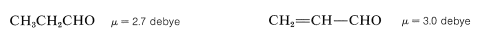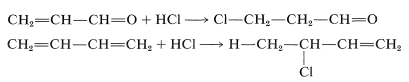# 17.6: α,β-Unsaturated Aldehydes and Ketones

$$\newcommand{\vecs}{\overset { \rightharpoonup} {\mathbf{#1}} }$$ $$\newcommand{\vecd}{\overset{-\!-\!\rightharpoonup}{\vphantom{a}\smash {#1}}}$$$$\newcommand{\id}{\mathrm{id}}$$ $$\newcommand{\Span}{\mathrm{span}}$$ $$\newcommand{\kernel}{\mathrm{null}\,}$$ $$\newcommand{\range}{\mathrm{range}\,}$$ $$\newcommand{\RealPart}{\mathrm{Re}}$$ $$\newcommand{\ImaginaryPart}{\mathrm{Im}}$$ $$\newcommand{\Argument}{\mathrm{Arg}}$$ $$\newcommand{\norm}{\| #1 \|}$$ $$\newcommand{\inner}{\langle #1, #2 \rangle}$$ $$\newcommand{\Span}{\mathrm{span}}$$ $$\newcommand{\id}{\mathrm{id}}$$ $$\newcommand{\Span}{\mathrm{span}}$$ $$\newcommand{\kernel}{\mathrm{null}\,}$$ $$\newcommand{\range}{\mathrm{range}\,}$$ $$\newcommand{\RealPart}{\mathrm{Re}}$$ $$\newcommand{\ImaginaryPart}{\mathrm{Im}}$$ $$\newcommand{\Argument}{\mathrm{Arg}}$$ $$\newcommand{\norm}{\| #1 \|}$$ $$\newcommand{\inner}{\langle #1, #2 \rangle}$$ $$\newcommand{\Span}{\mathrm{span}}$$

## Structure and Spectral Properties

The most generally useful preparation of $$\alpha$$,$$\beta$$-unsaturated carbonyl compounds is by dehydration of aldol addition products, as described in Section 17-3D. Conjugation of the carbonyl group and double bond has a marked influence on spectroscopic properties, particularly on ultraviolet spectra, as the result of stabilization of the excited electron states, which for $$\pi \rightarrow \pi^*$$ transitions can be described in terms of important contributions of polar resonance structures (see Sections 9-9B and 16-3B):Such resonance is much less important in the ground state but is still sufficiently important to account for the moderate differences in dipole moments between saturated and $$\alpha$$,$$\beta$$-unsaturated aldehydes and ketones; for example,The effect of conjugation also is reflected in infrared carbonyl frequencies (Section 16-3) and NMR spectra. With respect to the latter, it is found that the protons on the $$\beta$$ carbon of $$\alpha$$,$$\beta$$-unsaturated carbonyl compounds usually come at $$0.7$$ to $$1.7 \: \text{ppm}$$ lower fields than ordinary alkenic protons. The effect is smaller for the $$\alpha$$ protons.

There are many addition reactions of $$\alpha$$,$$\beta$$-unsaturated aldehydes, ketones, and related compounds that are the same as the carbonyl addition reactions described previously. Others are quite different and result in addition to the alkene double bond. Organometallic compounds are examples of nucleophilic reagents that can add to either the alkene or the carbonyl bonds of conjugated ketones (Section 14-12D). Hydrogen cyanide behaves likewise and adds to the carbon-carbon double bond of 3-butene-2-one, but to the carbonyl group of 2-butenal:All of these reactions may be classified as nucleophilic additions, but when addition occurs at the alkene bond, the orientation always is such that the nucleophile adds at the $$\beta$$ carbon. An example is the addition of methanol catalyzed by sodium methoxide:Nucleophilic reagents normally do not attack carbon-carbon double bonds (Section 10-6). The adjacent carbonyl function therefore must greatly enhance the reactivity of the double bond toward such reagents. This enhancement is not surprising when it is realized that the attack of a nucleophile produces a stabilized enolate anion:The products are formed from the enolate intermediate by proton transfer to either carbon or oxygen. If the proton adds to oxygen the enol is formed, which is unstable with respect to the ketone and ultimately will rearrange:Reactions of this type are referred to in a variety of terms, many of which are rather confusing and nondescriptive. They sometimes are classified as 1,4-additions, implying that addition occurs across the terminal protons of the conjugated system. A synonymous term is conjugate addition. When the nucleophile is a carbanion, the reaction is called a Michael addition. Thus, by this definition, Equation 17-7 represents a Michael addition. Another, perhaps more typical, example is the addition of an enolate to a conjugated ketone:Michael-type additions, like aldol additions, are useful for the formation of carbon-carbon bonds.

Electrophilic addition of hydrogen halides to $$\alpha$$,$$\beta$$-unsaturated aldehydes and ketones places the halogen on the $$\beta$$ carbon. This orientation is opposite to that observed for related additions to conjugated dienes: